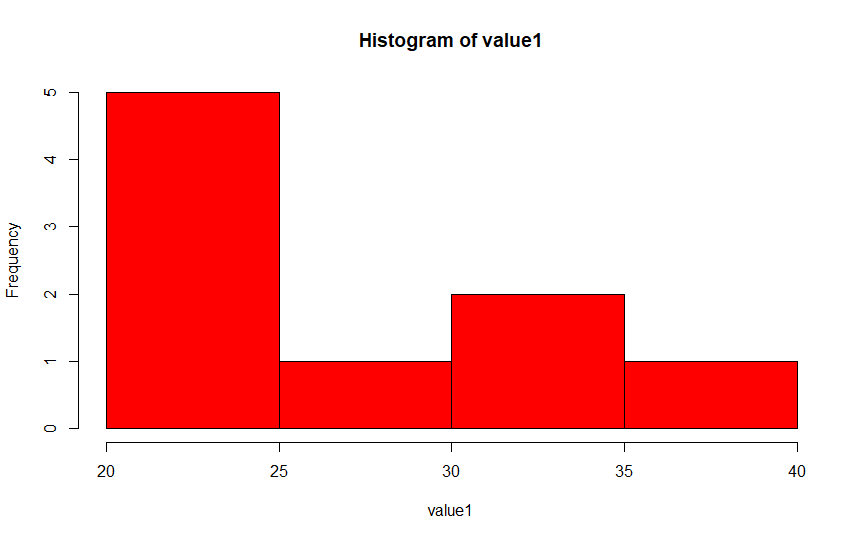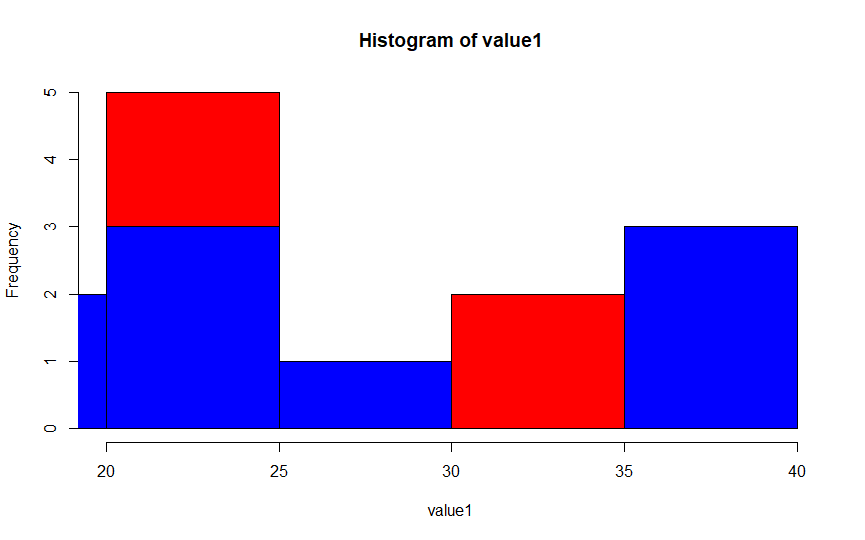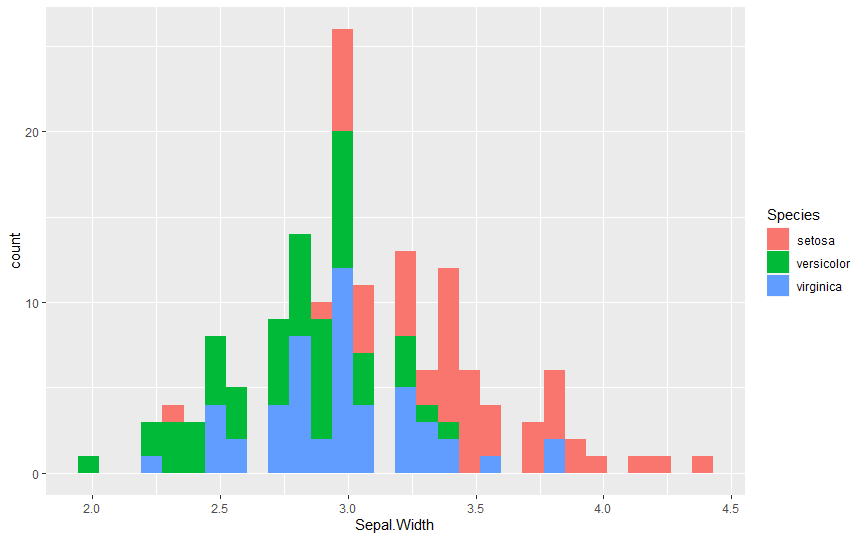# Create Stacked Histogram in R

Manav Narula Dec 30, 2020

We know R is considered to be a powerful programming language for data and statistical analysis. For this reason, R is equipped with many functions for different types of graphs and plots. Such plots are very useful and can provide good insights into the data.

One such graph available is the histogram. A histogram is used to plot a distribution with different bars. This tutorial will demonstrate how to create a simple histogram using the `hist()` function and will also cover stacked histograms with multiple populations using `hist()` and `ggplot()` functions.

The following code shows a simple histogram using the `hist()` function.

``````value1 = c(20,20,25,25,40,35,30,20,35)
hist(value1,col = "red")
``````A lot of other customizations can be added to the graph using different parameters available in the `hist()` function. We can also use `ggplot()` for the same purpose.

When dealing with stacked histograms, we have either two or more populations plotted on the same graph. We can do it in two ways, either we have two different variables that are to be plotted on the same graph, or we have one variable with different categories.

For the first approach, we will use the `hist()` function. The following code snippet explains how:

``````value1 = c(20,20,25,25,40,35,30,20,35)
value2 = c(15,25,30,25,25,20,40,40,40)

hist(value1,col = "red")
hist(value3, add = T, col = "blue")
``````In this method, we created a simple histogram and added the second graph to the first using the `add` parameter.

For the second approach, we will use a built-in sample dataset called `iris`. It contains the details of 3 plant species. We will plot the `Sepal.Width` column using the `ggplot()` function. We should load the `ggplot2` library to use the `ggplot()` function.

``````library(ggplot2)
ggplot(data=iris, aes(x=Sepal.Width,fill = Species)) + geom_histogram()
``````The `geom_histogram` specifies the plot type as a histogram. In the `ggplot()` function, we specify the variable to be plotted, and we color the histogram based on the categorical variable, `Species`.

Author: Manav Narula

Manav is a IT Professional who has a lot of experience as a core developer in many live projects. He is an avid learner who enjoys learning new things and sharing his findings whenever possible.